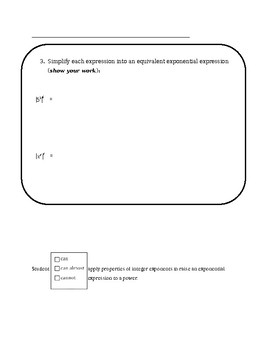DID YOU KNOW:
Seamlessly assign resources as digital activities

Learn how in 5 minutes with a tutorial resource. Try it Now

Learn More# 8th Grade CCLS Expressions and Equations Questions8th
Subjects
Standards
Resource Type
Formats Included
• Word Document File

### Description

In this packet, standards 8.EE.1, 8.EE.3, 8.EE.4, 8.EE.5, 8.EE.6, 8.Ee.7 and 8.EE.8 have been broken down into single question pieces (35 pages). It has never been simpler to assess students' strengths and weaknesses. Rather than complex multi-step problems, these tasks target each individual piece of the standard to determine and document what students can, can almost and cannot do.

Total Pages
N/A
Teaching Duration
N/A
Report this Resource to TpT
Reported resources will be reviewed by our team. Report this resource to let us know if this resource violates TpT’s content guidelines.

### Standards

to see state-specific standards (only available in the US).
Solve real-world and mathematical problems leading to two linear equations in two variables. For example, given coordinates for two pairs of points, determine whether the line through the first pair of points intersects the line through the second pair.
Solve systems of two linear equations in two variables algebraically, and estimate solutions by graphing the equations. Solve simple cases by inspection. For example, 3𝘹 + 2𝘺 = 5 and 3𝘹 + 2𝘺 = 6 have no solution because 3𝘹 + 2𝘺 cannot simultaneously be 5 and 6.
Understand that solutions to a system of two linear equations in two variables correspond to points of intersection of their graphs, because points of intersection satisfy both equations simultaneously.
Analyze and solve pairs of simultaneous linear equations.
Solve linear equations with rational number coefficients, including equations whose solutions require expanding expressions using the distributive property and collecting like terms.